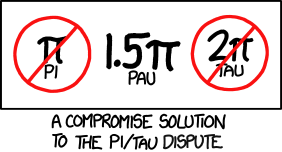## Pi vs. Tau

Happy pi day, y’all!Ahhhh pis, pies, how nice. Today’s theme is math. Math, math, and more math! I do like math (if it’s not a word problem. word problems will be the death of me) In honor of pi day, I’m posting the first hundred decimal places of pi:

#### 3.1415926535 8979323846 2643383279 5028841971 6939937510 5820974944 5923078164 0628620899 8628034825 3421170679 …

Neat, ain’t it? (did I just say “ain’t? oh-oh…) Click here if you want to see more digits! math.com has up to 200 million digits!!!

Now. I just learned something cool today. In math we were doing a pi day project and our teacher was playing pi videos in the background. Another kid in my class, let’s call him N, happens to be quite annoying and disruptive. However, he’s also really smart and knowledgable! (pretty sure he has bad grades though… my math teacher kinda hates him and she’s always saying “oh, N, just wait until you see your report card”. I feel bad for him 😦 even he’s not that bad)

But, I digress.

He was talking about how he doesn’t “celebrate” pi day because there’s something cooler called tau. Tau is 2pi and an easier way to calculate circumference (according to mathematicians). I know you may hate math, but I gotta show you- even I think it’s cool:

formula for calculating circumference of a circle:

c= 2r ord

Well, tau is 2 * pi, right? And in the formula you have pir, and… 2! So if you use this formula, it would also work:

c=r

which means that if you multiply tau and the radius of the circle, you’ll get the circumference! It’s more accurate (mathematically) because you use the radius to calculate most circle-related-things, not the diameter.

Here, at The Verge, Chaim Gartenberg explains:

Step back in time to when you first learned geometry and recall the simple origins: no matter what circle you’re using, if you divide the circumference of the circle by the diameter, you’ll get the same answer: an endless number, starting with the digits 3.14159265… (aka pi).

And right there is the fundamental flaw. The thing is, we don’t actually use diameter to describe circles. We use the radius, or one-half the diameter. The circle equation uses the radius, the area of a circle uses the radius, and the fundamental definition of a circle — “the set of all points in a plane that are at a given distance from a given point, the center” — is based on the radius. Plugging that into our circle constant equation gives us a new circle constant equivalent to 2π, or 6.28318530717… also known as tau.

Using the radius is more correct, which is why tau is there. If you want to learn more about tau, check out https://tauday.com/.

OOH. Lookie here~LOL our teacher showed this to our class… N totally freaked out. He is SERIOUSLY passionate about tau…

Did your mind explode yet? (Don’t worry- mine didn’t either. It’s cool, though, right?)

Eh. Maybe I’m just a weirdo who likes math. Anyway…

Happy Pi Day,